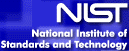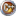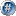# Case study: Ultrasonic Reference Block StudyNIST info: This example illustrates the construction of a non-linear regression model for ultrasonic calibration data. This case study demonstrates fitting a non-linear model and the use of transformations and weighted fits to deal with the violation of the assumption of constant standard deviations for the errors. This assumption is also called homogeneous variances for the errors. Full description can be found here.

Data: The ultrasonic reference block data consist of a response variable and a predictor variable. The response variable is ultrasonic response and the predictor variable is metal distance. These data were provided by the NIST scientist Dan Chwirut (avaiable here).

In this example we will follow the suggested steps, listed at NIST pages.

1) Read in the data: For non-linear regression we'll use tMtxNonLinReg control. First we have to create the control and populate it's X and Y vectors with data.

2) Plot the y=y(x) chart to get an idea about the fit model. For this, you can use the MtxVecTee.DrawValues method. These two steps can be acomplished by the following code class:``````Uses MtxVec, StatTools, Math387, MtxVecTee;

procedure FitModel;
var nlr: TMtxNonLinReg;
begin
nlr := TMtxNonLinReg.Create(nil);
try
DrawValues(nlr.X,nlrY,Chart1.Series);````````````#include "MtxVecCpp.h"
#include "StatTools.hpp"
#include "Math387.hpp"
#include "MtxVec.hpp"
#include "MtxVecTee.hpp"

void FitModel(void); {
TMtxNonLinReg* nlr = new TMtxNonLinReg(NULL);
Chart1->FreeAllSeries();
try {
DrawValues(nlr->X,nlr->Y,Chart1->Series,0,1,false);````````````using Dew.Math;
using Dew.Math.Units;
using Dew.Stats.Units;
using Dew.Stats;

namespace Dew.Tests
{
private void FitModel() {
TMtxNonLinReg nlr = new TMtxNonLinReg(null);tChart1.Series.Clear();
try {
MtxVecTee.DrawValues(nlr.X, nlr.Y, tChart1,0,1,false);
``````

The y=y(x) plot shows an exponentially decaying pattern in the data. This suggests that some type of exponential function might be an appropriate model for the data. As suggested, the Exp(-a*x)/(b+c*x) function is a good choice. To perform a non-linear fit, we'll have to define the regression function and starting values for regression routine. We will determine starting values based on a grid of the parameter values. As suggested, in this case te grid is 0.1 to 1.0 in increments of 0.1, where the best parameter values minimize residual sum of squares In this case we will also "vectorize" the regression function so that all y(x) values will be calculated in one go (speed gain for large sample sizes). Below is the implementation:``````// Scalar version => simpler, but slower for multiple x
function RegFun(const b: Array of TSample; x: TSample): TSample; overload;
begin
Result := Exp(-b*x)/(b+x*b);
end;

// Vector version => more complex, but faster for multiple x
var wrk1:Vector;
begin
// wrk1 = Exp(-B*x)
wrk1.Normalize(X,0.0,-1.0/B.Values);
wrk1.Exp;
// 1/(B+B*x)
Y.Copy(X);
Y.Mul(B.Values);
Y.Inv;
// Y = Exp(-B*x)/(B+B*x)
Y.Mul(wrk1);
end;
procedure InitialEstimates(X,Y: TVec; B: TVec);
i: Integer;
parval: TSample;
wrk1,Residuals,YCalc: Vector;
begin
wrk1.Size(b);
wrk1.SetZero;
b.Copy(wrk1);
// iterate for all parameters
for i := 0 to b.Length - 1 do
begin
// evaluate on interval [0,1], step = 0.1
parval := 0.0;
while (parval<=1.0) do
begin
wrk1.Values[i] := parval;
// evaluate function (vectorized version => faster)
RegFun(wrk1,X,YCalc);
Residuals.Sub(YCalc,Y);
parval := parval + 0.1;
begin
b.Copy(wrk1);
end;
end;
end;
end;````````````// Scalar version => simpler, but slower for multiple x
void double RegFun(const double* b, double x);
{
return Math::Exp(-b*x)/(b+x*b);
}
// Vector version => more complex, but faster for multiple x
void RegFun(Vector B,Vector X,Vector Y);
{
Vector wrk1;
// wrk1 = Exp(-B*x)
wrk1->Normalize(X,0.0,-1.0/B->Values);
wrk1->Exp();
// 1/(B+B*x)
Y->Copy(X);
Y->Mul(B->Values);
Y->Inv();
// Y = Exp(-B*x)/(B+B*x)
Y->Mul(wrk1);
}
void InitialEstimates(Vector X,Vector* Y, Vector B);
{
Vector residuals, wrk2, ycalc;
wrk1->Size(b);
wrk1->SetZero();
b->Copy(wrk1);
// iterate for all parameters
(for int i=0; iLength; i++)
{
// evaluate on interval [0,1], step = 0.1
parval = 0.0;
while (parval<=1.0)
{
wrk1->Values[i] = parval;
// evaluate function (vectorized version => faster)
RegFun(wrk1,X,ycalc);
residuals->Sub(ycalc,Y);
parval += 0.1;
{
b->Copy(wrk1);
}
}
}
}````````````// Scalar version
double RegFun(double[] b, double x)
{
return Math.Exp(-b*x)/(b+b*x);
}

// Vectorized version
void RegFun(TVec B, TVec X, TVec Y)
{
Vector wrk1 = new Vector(0);
// wrk1 = Exp(-B*x)
wrk1.Normalize(X,0.0,-1.0/B.Values);
wrk1.Exp();
// 1/(B+B*x)
Y.Copy(X);
Y.Mul(B.Values);
Y.Inv();
// Y = Exp(-B*x)/(B+B*x)
Y.Mul(wrk1);
}

void InitialEstimates(TVec X, TVec Y, TVec B)
{
Vector wrk1 = new Vector(0);
Vector residuals = new Vector(0);
Vector ycalc = new Vector(0);
wrk1.Size(B);
wrk1.SetZero();
B.Copy(wrk1);
for (int i=0; i<B.Length; i++)
{
parval = 0.0;
while (parval<=1.0)
{
wrk1.Values[i] = parval;
// evaluate function (vectorized version => faster)
RegFun(wrk1,X,ycalc);
residuals.Sub(ycalc,Y);
parval += 0.1;
{
B.Copy(wrk1);
}
}
}
}``````

3) Perform non-linear regression on data: Now that we have the regression function and initial estimates for regression coefficients, we can perform the actual non-linear regression.``````procedure FitModel;
var nlr: TMtxNonLinReg;
residuals: Vector;
begin
nlr := TMtxNonLinReg.Create(nil);
try
// Define and connect to regression function
nlr.B.Length := 3;
nlr.RegressFunction := RegFun;

// initial values for coefficients
InitialEstimates(nlr.X, nlr.Y,nlr.B);

// Perform non-linear regression
nlr.Recalc;
// Calculate residuals
residuals.Sub(nlr.YCalc,nlr.Y);
finally
nlr.Free;
end;
end;````````````void FitModel(void);
{
Vector residuals;
TMtxNonLinReg* nlr = new TMtxNonLinReg(NULL);
try
{
// connect to regression function
nlr->RegresFun = RegFun;

// initial estimates for coefficients
nlr->B->Length = 3;
InitialEstimates(nlr->X,nlr->Y,nlr->B);

// perform non-linear regression
nlr->Recalc();
residuals->Sub(nlr->YCalc,nlr->Y);
}
__finally
{
nlr->Free();
}
}````````````private void FitModel()
{
TMtxNonLinReg nlr = new TMtxNonLinReg(this);
Vector residuals = new Vector(0);
// connect to regression function
nlr.RegressFun += new Dew.Stats.TRegressFun(RegFun);
// initial values for coefficients
nlr.B.Length = 3;
InitialEstimates(nlr.X,nlr.Y,nlr.B);

// Perform non-linear regression
nlr.Recalc();
// Calculate residuals
residuals.Sub(nlr.YCalc,nlr.Y);
} ``````

## Additional things to try :

• Try transforming the y values before applying the non-linear regression. Suggested transformations: sqrt(y), ln(y), 1.0/y. This can be done with a single line of code class: nlr.Y.Sqrt();
• Perform a weigted regression on the same data. Define weights as specified at NIST pages.
• Plot data, fitted data and residuals by using DrawValues routine.
• Plot normal probability plot of residuals.
• Plot histogram of residuals.
• Compare the results with quoted results at NIST pages.